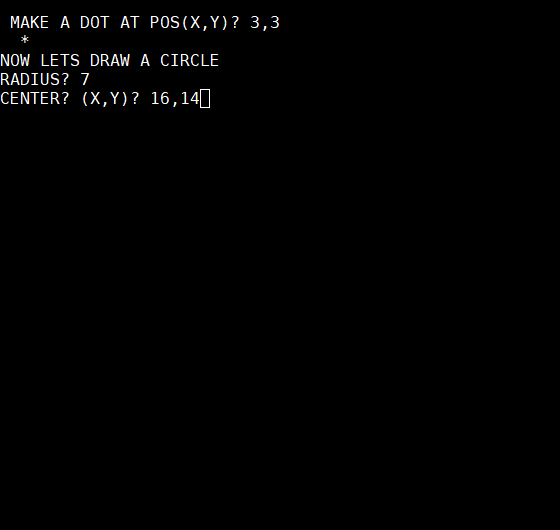# Terminal ESC Codes part 2

## positioning the cursor with esc terminal codes

The command to set the cursors pos in a terminal window is really simple:

``````(ESC)[x;yH
``````

where x and y are the coordinates from top left.

If you send this command, the corsor position changes immediately to x,y.

Problems with Kim-1 Basic:

number variables (not strings) are presented with a leading and ending space. Otherwise you could not read a line of integers and distinguish the single numbers (12345 could be 12 and 345 or 123 and 45).

But the terminal commands we send, must be precise. So we need to get rid of those spaces:

1. convert the integer into a string
2. cut the spaces off

``````X\$=STR\$(X):X\$=MID\$(X\$,2)
``````

Now we can insert our positions into the ‚move to‘ order.

``````PRINT ES\$+X\$+";"+Y\$+"H"+"*";
``````

A lot of commands for a simple ‚move to‘, a lot of dirtyhacks, but in the end you are able to adress any screen position.

Here's the example